Courses

# NCERT Solutions (Part- 4)- Algebraic Expressions and Identities Class 8 Notes | EduRev

## Mathematics (Maths) Class 8

Created by: Full Circle

## Class 8 : NCERT Solutions (Part- 4)- Algebraic Expressions and Identities Class 8 Notes | EduRev

The document NCERT Solutions (Part- 4)- Algebraic Expressions and Identities Class 8 Notes | EduRev is a part of the Class 8 Course Mathematics (Maths) Class 8.
All you need of Class 8 at this link: Class 8

Exercise 9.5

Question 1. Use a suitable identity to get each of the following products.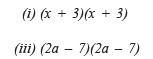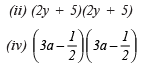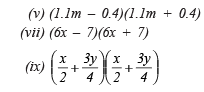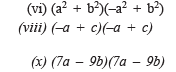Solution: (i) Using the identity,

(x + a)(x + b) = x2 + (a + b)x + ab

we have

(x + 3)(x + 3) = x2 + (3 + 3)x + (3 * 3)

= x2 + 6x + 9

(ii) Using the identity,

(x + a)(x + b) = x2 + (a + b)x + ab,

we have

(2y + 5)(2y + 5) = (2y)2 + (5 + 5)2y + (5 * 5)

= 4y2 + (10)2y + 25

= 4y2 + 20y + 25

(iii) Using the identity, (a – b)2 = a2 – 2ab + b2

(2a – 7)(2a – 7) = (2a – 7)2

= (2a)2 – 2(2a)(7) + (7)2

= 4a2 – 28a + 49

(iv) Using the Identity, (a – b)2 = a2 – 2ab + b2, we have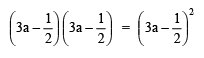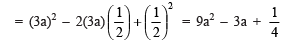(v) Using the identity, (a + b)(a – b) = a2 – b2,

we have

(1.1m – 0.4)(1.1m – 0.4) = (1.1m)2 – (0.4)2

= 1.21m2 – 0.16

(vi) ∵ (a2 + b2)(–a2 + b2) = (b2 + a2)(b2 – a2)

∴ Using the identity, (a + b)(a – b) = a2 – b2, we have

(b2 + a2)(b2 – a2)= (b2)2 – (a2)2 = b4 – a4

(vii) ∵ (6x – 7)(6x + 7) = (6x + 7)(6x – 7)

∴  Using the identity, (a + b)(a – b) = a2 – b2,

we have

(6x + 7)(6x – 7) = (6x)2 – (7)2

= 36x2 – 49

(viii) Using the identity, (a + b)2 = a2 + 2ab + c2, we have

(–a + c)(–a + c) = (–a + c)2

= (–a)2 + 2(–a)(c) + (c)2

= a2 + 2(–ac) + c2

= a2 – 2ac + c2

(ix) Using the identity, (a + b)2 = a2 + b2 + 2ab, we have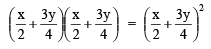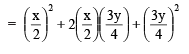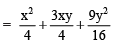(x) Using the identity, (a – b)2 = a2 – 2ab + b2 we have

(7a – 9b)(7a – 9b) = (7a – 9b)2

= (7a)2 – 2(7a)(9b) + (9b)2

= 49a2 – 126ab + 81b2

Question 2. Use the identity (x + a)(x + b) = x2 + (a + b)x + ab to find the following products.

(i) (x + 3)(x + 7) (ii) (4x + 5)(4x + 1) (iii) (4x – 5)(4x – 1)
(iv) (4x + 5)(4x – 1) (v) (2x + 5y)(2x + 3y)
(vi) (2a2 + 9)(2a2 + 5) (vii) (xyz – 4)(xyz – 2)

Solution: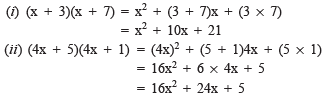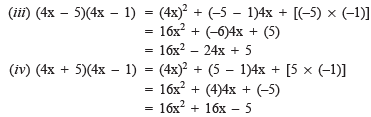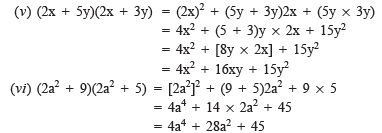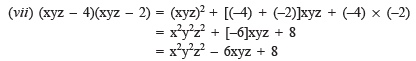Question 3. Find the following squares by using the identities.

(i) (b – 7) (ii) (xy + 3z)2 (iii) (6x2 – 5y)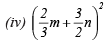(v) (0.4p – 0.5q)2  (vi) (2xy + 5y)2

Solution:

(i) (b – 7)2

Using (a – b)= a2 – 2ab – b2, we have

(b – 7)2 = (b)2 – 2(b)(7) + (7)2

= b2 – 14b + 49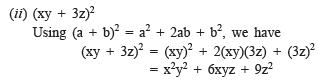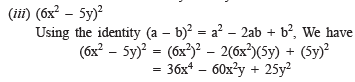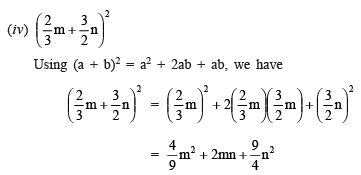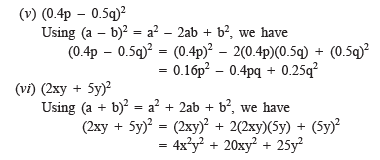Question 4. Simplify:

(i) (a2 – b2)2                                                        (ii) (2x + 5)– (2x – 5)2

(iii) (7m – 8n)2 + (7m + 8n)2                     (iv) (4m + 5n)2 + (5m + 4n)2

(v) (2.5p – 1.5q)2 – (1.5p – 2.5q)2            (vi) (ab + bc)2 – 2ab2c

(vii) (m2 – n2m)2 + 2m3n2

Solution:

(i) (a2 – b2)2 = (a2)2 – 2a2 * b2 + (b2)2 [using (a – b)2 = a2 – 2ab + b2]

= a4 – 2a2b2 + b4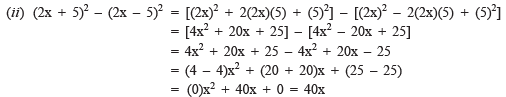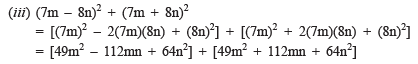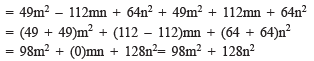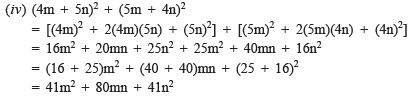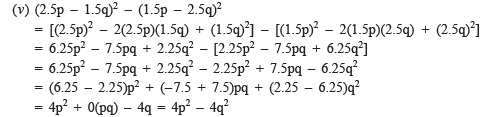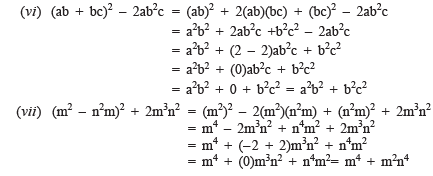Question 5. Show that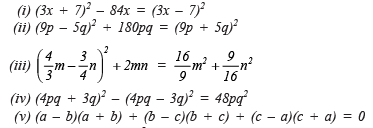Solution:

(i) LHS = (3x + 7)2 – 84x

= (3x)2 + 2(3x)(7) + (7)2 – 84x

= 9x2 + 42x + 49 – 84x

= 9x2 + (42 – 84)x + 49

= 9x2 – 42x + 49

RHS = (3x – 7)2

= (3x)2 – 2(3x)(7) + (7)2

= 9x2 – 42x + 49 = LHS

Since, LHS = RHS

∴ (3x + 7)2 – 84x = (3x – 7)2

(ii) LHS = (9p – 5q)+ 180pq

= (9p)2 – 2(9p)(5q) + (5q)2 + 180pq

= 81p2 – 90pq + 25q2 + 180pq

= 81p+ (–90 + 180)pq + 25q2

= 81p+ 90pq + 25q2

RHS = (9p + 5q)2

= (9p)2 + 2(9p)(5q) + (5q)2

= 81p2 + 90pq + 25q2

Since, LHS = RHS

∴ (9p – 5q)2 + 180pq = (9p + 5q)2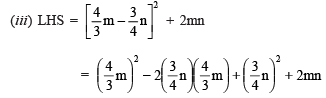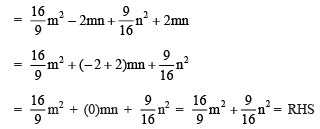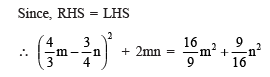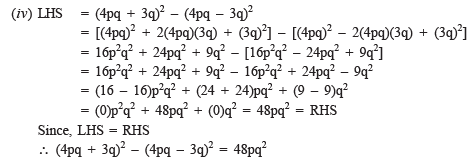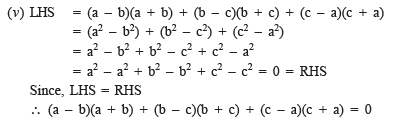Question 6. Using identities, evaluate:

(i) 712 (ii) 992 (iii) 1022 (iv) 9982 (v) 5.22 (vi) 297 * 303 (vii) 78 * 82 (viii) 8.92 (ix) 1.05 * 9.5

Solution:

(i) ∵ (71) = (70 + 1)

∴ (71)2 = (70 + 1)2

= (70)2 + 2(70)(1) + (1)2        [Using (a + b)2 = a2 + 2ab + b2]

= 4900 + 140 + 1 = 5041

(ii) We have 100 – 1 = 99

∴ (99)2 = (100 – 1)2

= (100)2 – 2(100)(1) + (1)2    [Using (a – b)2 = a2 – 2ab + b2]

= 10000 – 200 + 1 = 9801

(iii) ∵ 102 = 100 + 2

∴ (102)2 = (100 + 2)2

= (100)2 + 2(100)(2) + (2)2         [Using (a + b)2 = a2 + 2ab + b2]

= 10000 + 400 + 4 = 10404

(iv) ∵ 998 = 1000 – 2

∴ (998)2 = (1000 – 2)2

= (1000)2 – 2(1000)(2) + (2)2              [Using (a – b)2 = a2 – 2ab + b2]

= 1000000 – 4000 + 4 = 996004

(v) ∵ 5.2 = 5 + 0.2

∴  (5.2)2 = (5 + 0.2)2

= (5)2 + 2(5)(0.2) + (0.2)2                  [Using (a + b)2 = a2 + 2ab + b2]

= 25 + 2 + 0.04 = 27.04

(vi) ∵ 297 = 300 – 3 and 303 = 300 + 3

∴ 297 * 303 = (300 – 3)(300 + 3)

= (300)2 – (3)2                                [Using (a + b)(a – b) = a2 – b2]

= 90000 – 9 = 89991

(vii) ∵ 78 = 80 – 2 and 82 = 80 + 2

∴ 78 * 82 = (80 – 2)(80 + 2)

= 802 – (2)2                                   [Using (a + b)(a – b) = a2 – b2]

= 6400 – 4 = 6396

(viii) ∵ 8.9 = (9 – 0.1)

∴ (8.9)2 = (9 – 0.1)

= (9)2 – 2(9)(0.1) + (0.1)2              [Using (a – b)2 = a2 – 2ab + b2]

= 81 – 1.8 + 0.01

= 81.01 – 1.80 = 79.21

(ix) ∵ 1.05 = 1 + 0.05

∴ (1.05) * 9.5 = (1 + 0. 05) * 9.5

= (1 * 9.5) + (9.5 * 0.05)

= 9.500 + 0.475 = 9.975

Question 7. Using a2 – b2 = (a + b)(a – b), find:

(i) 512 – 492 (ii) (1.02)– (0.98)2 (iii) 1532 – 1472 (iv) 12.12 – 7.92

Solution:

(i) 512 – 492 = (51 + 49)(51 – 49)

= (100)* (2) = 200

(ii) (1.02)2 – (0.98)2 = (1.02 + 0.98)(1.02 – 0.98)

= (2.0) * (0.04) = 0.08

(iii) 1532 – 1472 = (153 + 147)(153 – 147)

= (300) * (6) = 1800

(iv) (12.1)2 – (7.9)2 = (12.1 + 7.9)(12.1 – 7.9)

= 20 * 4.2 = 84

Question 8. Using (x + a)(x + b) = x2 + (a + b)x + ab,
find: (i) 103 * 104 (ii) 5.1 * 5.2
(iii) 103 * 98 (iv) 9.7 * 9.8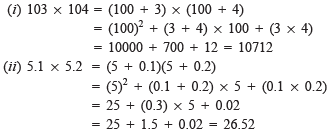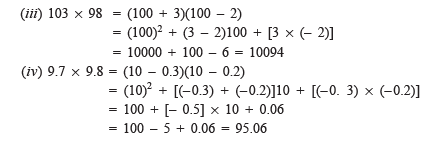## Mathematics (Maths) Class 8

212 videos|147 docs|48 tests

,

,

,

,

,

,

,

,

,

,

,

,

,

,

,

,

,

,

,

,

,

;